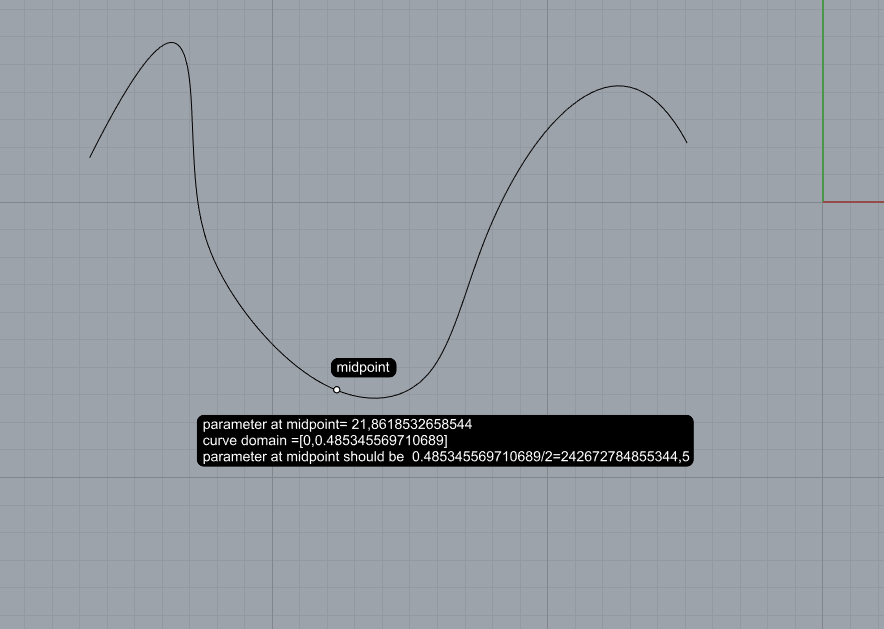# Curve closest point parameter error

Hi All. Rhino 6 c# rhinocommon last release 6.14.
I think there is a problem with parameter of Curve closest point
double t;
curve.ClosestPoint(point, out t);
In the attachment you can see that “t” evaluated in the middle point of the curve is not the middle of its domain.Sorry , I wrote in a wrong way (in the shot )the maximum of the domain: is not 0,48… but 48,…but you can understand the real problemYou can test it in the following file
closestpoint.3dm (169.1 KB)

this is correct, midpoint is not necessarily midpoint of the domain

1 Like

So if it is correct I cannot find the following . from curve closest point parameter i get a normalized parameter that is not correct because it is not corresponding to 0,5 of the curve. how can I get the normalized parameter of current point from a get point?

no you get the parameter, not the normalized parameter

but what do yo want to do, what’s your end goal?

1 Like

See these curve methods:

For example…

``````import Rhino
import rhinoscriptsyntax as rs

curve_id = rs.GetObject("Select curve", rs.filter.curve)
curve = rs.coercecurve(curve_id)
rc, t = curve.NormalizedLengthParameter(0.5)
t = curve.Domain.Mid
``````

– Dale

1 Like

My goal is to get normalized parameter from the current point of the dynamic method of a getpoint with constraint to a curve.

This should do it.

``````public class TestGetNormalizedCurvePoint : GetPoint
{
private Curve m_curve;

public TestGetNormalizedCurvePoint(Curve curve)
{
m_curve = curve;
Constrain(m_curve, false);
}

protected override void OnMouseMove(GetPointMouseEventArgs e)
{
if (null != m_curve && m_curve.ClosestPoint(e.Point, out double t))
{
var n = m_curve.Domain.NormalizedParameterAt(t);
RhinoApp.WriteLine(\$"Point: {e.Point.ToString()}");
RhinoApp.WriteLine(\$"  Parameter: {t.ToString()}");
RhinoApp.WriteLine(\$"  Normalized: {n.ToString()}");
}
}
}
``````

– Dale

1 Like

Thank you very much Dale, you hit the point and I think you can try to find the error that I found doing that. It is exactly what I did with a getpoint instead of a subclass. Now, if the parameter " t" is not at the middle of the domain , as Gijs said

and the mouse is on that point and the method that you use to normalize it m_curve.Domain.NormalizedParameterAt(t) is only a math operation , you will get a wrong normalized parameter, don 'you think? It is exactly my first question.
How can i get the correct normalized parameter 0,5 form m_curve.ClosestPoint(e.Point, out double t) if you put the mouse on the middle point? the middle point is obviously a test point to understand that the normalized parameter you will get in that way is not correct. I think the method to normalize the parameter should do something like this: Math. Abs(t-Domain. Min) /Math. Abs(Domain. Max-Domain. Min)? Am i wrong? thank you for your attention and timely response

Normalized parameter doesn’t mean that the midpoint of the curve will be at t==0.5 either

It just means your domain is mapped to a normalized domain 0-1

1 Like

Then there is some concept to make clearer. If you try to put a point with normalized length on 0.5